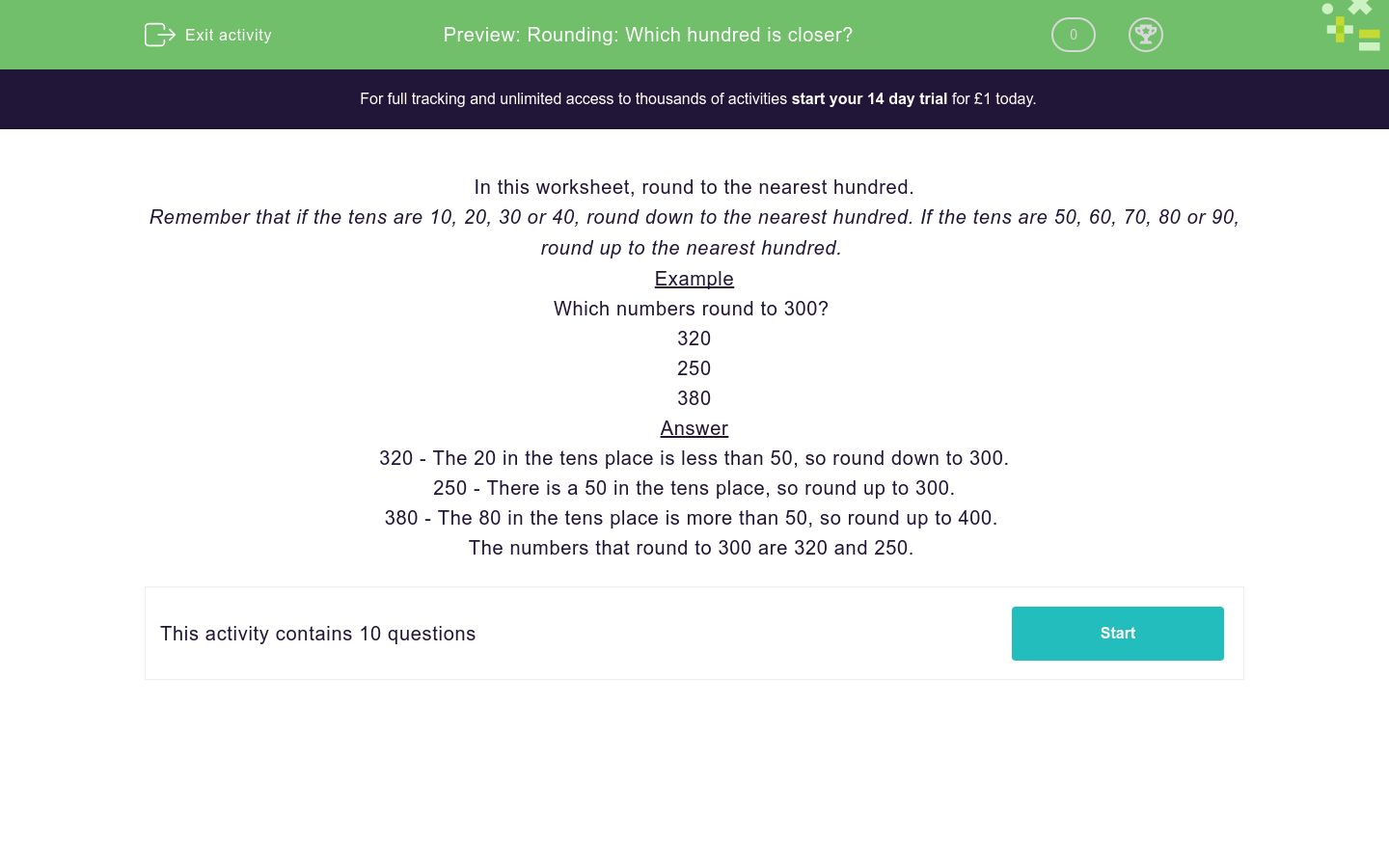# Rounding: Which hundred is closer?

In this worksheet, students round numbers to the nearest hundred.Key stage:  KS 2

Curriculum topic:   Number: Number and Place Value

Curriculum subtopic:   Round Numbers Up/Down (to 10, 100 or 1000)

Difficulty level:### QUESTION 1 of 10

In this worksheet, round to the nearest hundred.

Remember that if the tens are 10, 20, 30 or 40, round down to the nearest hundred. If the tens are 50, 60, 70, 80 or 90, round up to the nearest hundred.

Example

Which numbers round to 300?

320

250

380

320 - The 20 in the tens place is less than 50, so round down to 300.

250 - There is a 50 in the tens place, so round up to 300.

380 - The 80 in the tens place is more than 50, so round up to 400.

The numbers that round to 300 are 320 and 250.

Choose the numbers that round to 700.

701

710

750

Choose the numbers that round to 200.

248

205

237

Choose the numbers that round to 600.

248

610

737

Choose the numbers that round to 100.

112

152

111

Choose the numbers that round to 500.

510

560

502

Choose the numbers that round to 800.

851

806

838

Choose the numbers that round to 300.

301

353

349

Choose the numbers that round to 400.

401

351

449

Choose the numbers that round to 200.

401

199

301

Choose the numbers that round to 800.

840

480

850

• Question 1

Choose the numbers that round to 700.

701
710
EDDIE SAYS
701 - There are no tens, so round down to 700. 710 - The 10 in the tens place is less than 50, so round down to 700.
• Question 2

Choose the numbers that round to 200.

248
205
237
EDDIE SAYS
248 - The 40 in the tens place is less than 50, so round down to 200. 205 - There are no tens, so round down to 200. 237 - The 30 in the tens place is less than 50, so round down to 200.
• Question 3

Choose the numbers that round to 600.

610
EDDIE SAYS
610 - The 10 in the tens place is less than 50, so round down to 600.
• Question 4

Choose the numbers that round to 100.

112
111
EDDIE SAYS
112 - The 10 in the tens place is less than 50, so round down to 100. 111 - The 10 in the tens place is less than 50, so round down to 100.
• Question 5

Choose the numbers that round to 500.

510
502
EDDIE SAYS
510 - The 10 in the tens place is less than 50, so round down to 500. 502 - There are no tens, so round down to 500.
• Question 6

Choose the numbers that round to 800.

806
838
EDDIE SAYS
806 - There are no tens, so round down to 800. 838 - The 30 in the tens place is less than 50, so round down to 800.
• Question 7

Choose the numbers that round to 300.

301
349
EDDIE SAYS
349 - The 40 in the tens place is less than 50, so round down to 300. 301 - There are no tens, so round down to 300.
• Question 8

Choose the numbers that round to 400.

401
351
449
EDDIE SAYS
351 - There is a 50 in the tens place, so round up to 400. 401 - There are no tens, so round down to 400. 449 - The 40 in the tens place is less than 50, so round down to 400.
• Question 9

Choose the numbers that round to 200.

199
EDDIE SAYS
199 - The 90 in the tens place is more than 50, so round up to 200.
• Question 10

Choose the numbers that round to 800.

840
EDDIE SAYS
840 - The 40 in the tens place is less than 50, so round down to 800.
---- OR ----

Sign up for a £1 trial so you can track and measure your child's progress on this activity.

### What is EdPlace?

We're your National Curriculum aligned online education content provider helping each child succeed in English, maths and science from year 1 to GCSE. With an EdPlace account you’ll be able to track and measure progress, helping each child achieve their best. We build confidence and attainment by personalising each child’s learning at a level that suits them.

Get started